# Construction and Study of a Model of Oil Displacement by Water from the Reservoir

Construction and Study of a Model of Oil Displacement by Water from the Reservoir
Department of Computational Sciences and Statistic, Al Farabi Kazakh National University, Al Farabi, 74, Almaty 050040, Republic of Kazakhstan

Department of Mathematics, K. Zhubanov Aktobe Regional University, 34 A. Moldagulova Ave., Aktobe 030000, Republic of Kazakhstan

Corresponding Author Email:
alla.mussina@mail.ru
Page:
463-468
|
DOI:
https://doi.org/10.18280/mmep.100211
31 January 2023
|
Revised:
9 March 2023
|
Accepted:
15 March 2023
|
Available online:
28 April 2023
| Citation

OPEN ACCESS

Abstract:

In this paper, we investigated a two-phase model of incompressible fluid filtration. We apply the irregular grid method and the variable direction scheme. This approach gives the effect of solution accuracy near discontinuities (wells) due to the use of an irregular grid. The model is built taking into account the influence of capillary pressure and gravitational forces. The results confirm that the amount of oil that came out of the layer in a certain time is equal to the volume of water injected, except for the amount of water in the outlet stream in the same time. The proposed solutions of the new approach are intended to improve the methods and schemes of numerical investigation of this model. A balanced monotonic finite-difference scheme was developed and an efficient algorithm for its implementation was proposed. From a practical point of view, numerical modeling allows early prediction of performance. Thus, the applied aspect of the use of the obtained scientific result is the possibility of improving the process by taking into account the distribution of water saturation in the layer.

Keywords:

two-phase model, capillary pressure, oil displacement, free (unknown) boundaries

1. Introduction

The goal of this work is to consider the process of water and oil filtration through the reservoir of an oil deposit. The adjacent formations of the reservoir, being the third phase, have constant characteristics, that is, they are a constant. The purpose of mathematical modeling, as a rule, is to determine such optimal parameters of oil production, which would help to develop control decisions. This is primarily due to the high cost of conducting full-scale experiments, and it is also due to the large number of different parameters affecting the final results. With the development of computational tools, a certain scheme of calculation of multiphase fluid filtration problems has developed, that is, from a mathematical model to a numerical algorithm, and to the analysis of the results and further to the prediction.

The monograph by Konovalov  is the main scientific work considering the spectrum of problems related to the mathematical modeling of multiphase filtration. Emphasis is placed on an inseparable triad: a model - an algorithm - computer code. The monograph pays more attention to the splitting method in terms of the physical processes, the method of fictitious domains, and the method of front separation in a grid solution.

The Buckley-Leverett model is the most used model. It assumes equality of phase pressures. In this model, generalized Darcy laws, equations of state, and mass conservation laws are written for each phase.

This model has been well studied in the works of such scholars as Konovalov et al. . In this case, the equation for saturation in this model is a nonlinear hyperbolic partial derivative equation. It is characterized by gaps in the solution . We can say that the only way to get a prediction is with numerical methods. And difference schemes are often used. In this case, the problem arises of selecting schemes that are good at conveying the qualities of the solution. In the research of Baikov et al. , the Buckley-Leverett model for a one-dimensional model of two-phase filtration in porous medium was considered.

This level was reached in the study of the model, which takes into account capillary forces.

The problem of oil displacement with water was originally posed in the works by Leibenzon , and it assumed complete displacement of oil. This "piston" model is also used at present. However, numerous later studies have shown that water does not displace oil completely and a large zone is formed where both phases move together.

The second well-known filtering model is the Masket-Leverett model. Capillary forces are taken into account in this model. Laplace’s law is added to the system of equations. The model was investigated by Meirmanov et al. .

The equation for saturation in this model is a quasi-linear partial derivative equation of the parabolic type. According to the studies of Antontsev et al. , the conversion of relative phase permeabilities to zero, leads to the fact that the system becomes degenerate.

The main problem is described by Henry , an application of the IMPES method. Reservoir modeling is performed using numerical models that use digital machines to solve mathematical equations governing the behavior of fluids in porous media using a gridded format, which can accommodate the reservoir. It leads to getting an insight into the behavior of physical processes.

In 1986, Zhou  proposed a new method called the differential transformation method (DTM), which is considered to be one of the best numerical methods used to solve both ordinary and partial differential equations.

At present, the methods for the numerical solution of moving boundary problems can be conditionally divided into two groups: shock-capturing methods and explicit edge detection methods. It should be noted that shock-capturing methods provide a means of solving problems of a multiphase and multidimensional problem of thermal conductivity, but they are not suitable for solving practical problems and have low accuracy in determining the position of free boundaries. Edge detection schemes usually have good accuracy, but are algorithmically cumbersome and unsuitable for solving free (indeterminate) boundary problems.

One of the most accurate shock-capturing schemes is built using the finite element method (FEM). It is known that such a scheme has a low order of approximation near free (indeterminate) boundaries.

On the other hand, not only the gradient and the solution itself have discontinuities at the phase boundaries in multiphase diffusion problems. A simple change of variables often makes it possible to remove the solution gaps and use some of the shock-capturing methods. In the study of Escandon-Panchana et al. , methods of petroleum engineering were investigated together with information provided by geology and geophysics to quantify the reserves and identify production mechanisms that predict a reservoir's behavior.

However, for problems of non-isothermal diffusion, none of the known methods of this type is applicable [10-12].

The present study considers a two-phase incompressible fluid filtration model that accounts for capillary pressure. The model takes into account the influence of capillary pressure and gravitational force. Under certain conditions, capillary forces in the model play a significant role, and in some cases, the role of capillary effects is decisive.

In this paper, the research scheme includes the derivation of the mathematical model, the problem statement, and the algorithm of the numerical method for solving the problem. A balanced monotonic finite difference scheme is developed.

2. Problem Setting

The present study considers the problem in a two-dimensional formulation. The formation is considered to be homogeneous and thin. Approximation of equations Masket-Leverett is solved in two stages. In the first step, the hyperbolic equation for water saturation is solved. This equation describes the movement of the displacement fluid. Here, an explicit finite difference scheme of the 4th order of accuracy is used. In the second stage, a parabolic equation for water saturation is solved. This equation describes the action of capillary forces. The proposed approach is known as the physical splitting method process.

The oil displacement by water is a physical process. An increase in pressure, and water saturation increment are also caused by water injection in wells. It can be pointed out that the main problems are associated, first, with the nonlinearity of the obtained systems of equations.

In that case, we will denote porosity by m, densities of water and oil phases by $\rho_i$, dynamic viscosities will be designated as $\mu_i$ (i = 1 is for oil, i = 2 is for the water phase). They do not depend on the variables t, x, z.

Let the solution to the two-phase filtration problem be sought in a two-dimensional XZ region $\Omega=\left[0, R_x\right] x\left[0, R_z\right]$ with a boundary γ. One of the most common methods for the numerical solution of problems related to the two-phase filtration of incompressible fluid is the grid method.

Simultaneously, there is a transition from the areas of determining the solution to the original problem to the areas of the discrete grid. Accordingly, the functions of the discrete argument with the domains of definition replace the functions of the continuous argument.

The difference equations are replaced by differential equations of the original problem, and the same is applied to the limiting conditions of the original problem. As a result, for each problem, a closed system of linear (or nonlinear) algebraic equations is obtained, the solution to which is taken as an approximate solution to the original differential problem.

The outer boundary of the area is $\gamma=\partial \Omega=\gamma_0 \cup \gamma_1 \cup \gamma_2\left(\gamma_1 \cap \gamma_2=\varnothing\right)$. On one of the boundaries γ1, which has a length R1, let us set the input flow Q [m2/s], the output flow on the other boundary γ2 with a length R2 is defined by Q. At the boundary γ0 let us put a no-leak condition (Figure 1).

## 1.png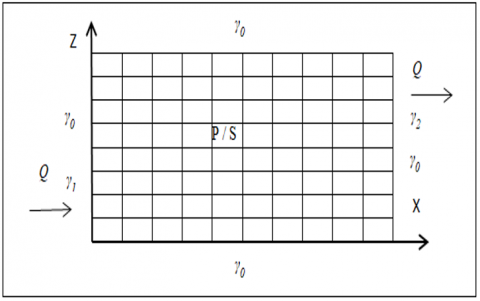Figure 1. Computational domain

Next, we will use the following ratios:

1) the continuity equation of our i -th phase will be written in the form:

## q1.png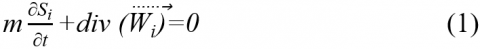2) generalized Darcy’s law:

$\vec{W}_i=-k_0 \frac{f_i(S)}{\mu_i} \nabla\left(\phi_i\right)=-k_i(S)\left(\nabla P_i\right)+\rho_i g \overrightarrow{e_2}$,

where,

Phase potentials if the z-axis is directed against gravity, can be written as:

$\phi_i=P_i+\rho_i g z$

Let the porous medium initially be filled with any one fluid. Let us bring another fluid to the surface of such a medium. The supplied fluid as if spreading over the surface of the pores, will tend to penetrate the porous medium, which is filled with the first fluid. It will displace the first fluid.

Therefore, a static equilibrium in a porous medium filled with two different immiscible fluids is possible precisely due to the presence of a capillary jump. The magnitude of this jump depends on the degree of insertion of the second fluid into the porous medium filled with the first fluid. Therefore, capillary pressure can be considered a function of the nano-saturation of the displacement phase:

$P_1-P_2=P_k(S)$

Capillary pressure, and also relative phase permeabilities are experimental values. Si is the saturation of the i-th phase, from the determination of water saturation, we obtain S1+S2 =1, $\vec{W}_i$ is the filtration rate of some i-th phase, m is the porosity of the layer. Let S = S2, S1=1-S; index 1 will be attributed to oil, index 2 will be attributed to water, then k0 is the permeability coefficient, let fi(S) be the relative phase permeabilities, which characterize the dependence of fluid filtration rate on water saturation, let μi be the coefficient of dynamic viscosity, $\overrightarrow{e_2}=(0,1)^T$; Pk(S) is capillary pressure. Capillary pressure and relative phase permeability are experimentally measured functions of water saturation.

Phase potentials if the z-axis is directed against gravity, can be written as:

$\phi_i=P_i+\rho_i g z$

Then

$\vec{W}_i=-k_0 \frac{f_i(S)}{\mu_i} \nabla\left(\phi_i\right)=-k_i(S)\left(\nabla\left(P_i\right)+\rho_i g \vec{e}_2\right)$

Let us determine that 0<Sн<Sb<1.

In this case, f1(S)=0 given that Sb<S<1, and f2(S)=0, when 0≤S≤SH, we get:

$k(S)=k_1(S)+k_2(S) \geq k_H>0,0 \leq S \leq 1$

$\overrightarrow{W_2}=-k_2(S) \nabla(P)-k_2(S) \rho_2 g \overrightarrow{e_2}$

$\overrightarrow{W_1}=-k_1(S)\left(\nabla(P)-P_s(S) \nabla S+\rho_1 g \overrightarrow{e_2}\right)$

## q2.png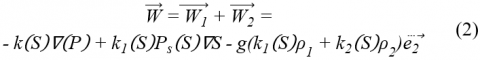Let k(S) = k1(S)+k2(S). Then we can add (1) and (2). And in this case, we get an elliptic equation, which defines the pressure:

$-\operatorname{div}(k(S)) \nabla P=-\operatorname{div}\left(k_1(S) P_s / \nabla S\right)+g\left(\rho_1 \frac{\partial k_1(S)}{\partial z}+\rho_2 \frac{\partial k_2(S)}{\partial z}\right)$                 (3)

To determine water saturation, we derive a parabolic equation:

$m \frac{\partial S}{\partial t}-\operatorname{div}\left(k_2(S) \nabla P\right)-g \rho_2 \frac{\partial k_2(S)}{\partial z}=0$                     (4)

The fluid flow at the inlet and outlet is Q. At the inlet we have only water phase: Q2in = Q, the oil phase is Q1in = 0.

The outlet will be both water and oil. At the outlet the water flow in the mixture is Q2out = Qk2(s)/k(s), the oil flow is Q1out = Qk1(s)/k(s).

Let us define boundary conditions of the second kind for Eq. (3):

## q5.pngFor the parabolic Eq. (4) we define the following boundary conditions:

## q6.png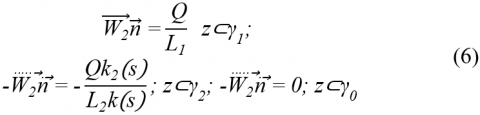Initial data P(0,x,z) = 0, S(0,x,z) = S0(x,z).

To determine the pressure P, the elliptic Eq. (3) with boundary conditions of the second kind (5), and for determination of water saturation S - parabolic Eq. (4) with boundary conditions (6) and initial data

$P(0, x, z)=0, S(0, x, z)=S^0(x, z)$

Eq. (3) has a nonunique solution, and Eq. (4) turns out to be an equation with a degenerate diffusion coefficient.

The problem (3), (4) with boundary conditions (5) and (6) had to be solved.

Limit saturation values of the displacement phase are SHand SBsuch that at S≤SHand S≥SBthe corresponding phase permeability fi(S) tends to zero. It follows from Darcy's generalized law that if S≤SH, then the displacing phase is stationary, if S≥SB, then the displaceable phase is stationary. Here, SH is called residual water saturation, 1- SB residual oil saturation.

3. Solution Algorithm and Numerical Results

We divided the calculation area into cells. On the x-axis, we divide the area by lines ihx,i=0...p, on the y-axis, divide the area by lines $j h_z$ j=0...d, then hx=Rx/p, hz=Rz/d. Nodes to calculate xi=(i-1/2)hx, I =1..p, zj=(j-1/2)hz, j=1..d. The volume of the calculation cell is equal to $V_h=h_x h_z, S^0(x, z)=0.2$.

By integrating Eqns. (4) and (6), we built a difference scheme.

$\frac{1}{V_h} \int_{V_h} d V$

Let R1 = R2 = hz, j1 is the number for the input cell, j2 is the output cell. The implicit system of equations is iterated:

$s^{n, 0}=s^{n-1}, p^{n, 0}=p^{n-1}$

Then at some step n we could switch to some system of equations, which later by iterations we reduced to the form:

$A\left(s^{n, k}\right) p^{n, k+1}=B\left(s^{n, k}\right) s^{n, k}+Q /\left.V_h\right|_{j 1}-Q /\left.V_h\right|_{j 2}$                 (7)

$s^{n, k+1}=s^{n-1}-\lambda T\left(s^{n, k}\right) p^{n, k+1}+\left.\lambda\left(Q / V_h\right)\right|_{j 1}-\frac{k_2\left(s^{n, k}\right)}{k\left(s^{n, k}\right)} Q /\left.V_h\right|_{j 2}$                      (8)

Matrices A, B, and C are symmetric and positively semi-defined. Eq. (7) at each iteration step according to s, is solved iteratively using the peremptory method of core gradients.

A symmetric positional fully semi-defined matrix R, corresponding to a five-point approximation, is taken as a re-conditioner of the Laplace operator in a rectangle with boundary conditions of the second kind. As ker A=ker R, the solution to the problem (7) lies in the im A subspace. After graduation iterations to highlight a single solution are renormalized.

To solve this problem, we used the split-variable method using the Fourier transform. The split-step (Fourier) method provides an excellent methodology to solve time-dependent partial differential equations. This method is ubiquitously used in engineering and physics applications . Splitting methods are frequently recommended for the integration of (nonlinear) equations due to their fast computability and high accuracy. Furthermore, they preserve the geometric features of the exact solution. The exponential Fourier transform method has been used in the research of Ike .

The theoretical justification of the split-variable method by physical processes for real nonlinear problems remains open. Konovalov provides a justification for the split-variable method by physical processes using a computational experiment . In a rectangle with sides Rx, Rz, a solution to the system of equations is sought that satisfies the initial and edge conditions. The solution of the two problems was described. In the first problem, the phase pressure is set on the boundary. In the second task, the filtration area is enclosed in an impermeable formation, and inside the filtration area, there are injection and production wells with specified flow rates. It was determined R=100. The number of calculated points in the tasks was 100. A comparison of the difference schemes was made and recommendations were prepared for using the split-variable method to calculate the filtration tasks of the two-phase non-compressible fluid taking into account capillary forces.

The following values of the physical parameters were used in numerical calculations: calculations were performed in a rectangle with sides Rx, Rz, -Rx=100 m, Rz=25 m, water entry was made in the cell x=0.0, z=0.0, or x=0.0, z=Rz, and oil recovery - in the cell x=Rx, z=Rz.

Calculations were performed at hx, hz=1 m, R1, R2=hz, the water flow rate varied from 1E-01 to 1E-04, m=0,5. Water consumption varied from 1E-1 to 1E-4. Total volume of oil in the layer Vf=1E-3m2.

The following characteristics will be used for the model:

$K_0=3.06 E-12 \mathrm{~m}^2, \mu_1=9.28 E-04 \frac{\mathrm{kg}}{\mathrm{m}} *_{s,} \mu_2=1.15 E-04 \frac{\mathrm{kg}}{\mathrm{m}} * s$

The following formulas were used for the relative phase permeabilities:

$f_1(S)= \begin{cases}1 & \text { if }\left(0<S \leq S_H\right) \\ \left(\frac{S_B-S}{S_B-S_H}\right) & \text { if }\left(\underline{S} \leq S \leq S_B\right) \\ 0 & \text { if }\left(S_B \leq S<1\right)\end{cases}$

$f_2(S)= \begin{cases}0 & \text { if }\left(0<S \leq S_H\right) \\ \left(\frac{S-S_H}{S_B-S_H}\right) & \text { if }\left(S_H \leq S \leq S_B\right) \\ 1 & \text { if }\left(S_B \leq S<1\right)\end{cases}$

$S_B=0.8$

$S_H=0.2$

From these relations, from (4), the boundary condition (5), and the initial conditions, we obtain the relation:

$m \int_{\Omega}\left(S(t, x, y)-S^0(0, x, y)\right) \partial \Omega=Q t-\frac{Q}{L_2} \int_0^t \int_{\gamma_2} \frac{k_2\left(S\left(t^{\prime}\right)\right)}{k\left(S\left(t^{\prime}\right)\right)} \partial \gamma_2 \partial t^{\prime}$             (9)

The amount of oil that came out of the layer in some time t is equal to Vp(t). It is equal to the volume of water that was pumped and minus the water that was formed in the outlet stream during the same time.

Figure 2 shows the distribution of water and oil. The X-axis shows the fraction of injected water Qt/Vf, the Y-axis shows the fraction of released oil Vp(t)/Vf relative to the total oil reserve in the layer.

## 2.png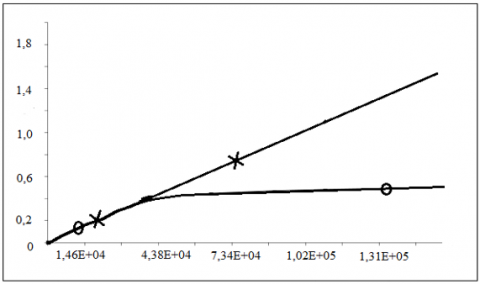Figure 2. The proportion of injected water (line X) and released oil (line O)

The resulting graph shows that at first the shares of water and oil in the flow coincide. Furthermore, we can see that the share of oil becomes constant. Water breakthrough occurs when it approaches the 0.4 mark.

As a result, it turned out that the time distribution of water saturation in the layer is practically independent of whether water input occurs in the cell (x=0.0, z=0.0) or in the cell (x=0, z=Rz) (water output is always in the cell (x=Rx, z=Rz). First there is the filling of the layer near the line x=0, and only then the water moves along the bottom (z=0). And then the water breaks through to the exit point (Figure 3).

## 3.pngFigure 3. Distribution of water saturation in the layer

One of the methods to increase oil recovery is waterflooding of oil objects. Water pumped into the reservoir performs two functions: maintaining reservoir pressure and displacing oil to the bottoms of producing wells.

The distribution of water saturation and oil saturation in this case, due to the heterogeneity of the reservoir, is significantly different in different areas of the development site. This leads to residual unexploited oil reserves. Some models allow us to estimate the distribution of these residual stocks. Models are based on quantifying such saturation coefficients.

The peculiarity of this studied problem is that the computational algorithms were built based on the known results of works of Kaliev and Sabitova , and after that the time derivatives of water saturation were added. In the study of this mathematical model, qualitative properties of solutions were also investigated: asymptotic behavior with unlimited time increase, periodicity in time, and automodelicity in the two-dimensional case. These properties play an important role in the construction of computational algorithms and numerical implementation of specific tasks using computers.

4. Discussion

Scientific novelty: possible variants of model solvability are shown, numerical model of Masket-Leverett supplemented with consideration of water and oil sources is implemented and experimentally substantiated. The results were tested with real technological indicators of the oil and gas field in the Atyrau region of the Republic of Kazakhstan.

For analysis, control and forecast calculations it is quite possible to use the results.

In the present work, we determined the pressure from elliptic Eq. (3) with boundary conditions of the second kind (5) and water saturation S from parabolic Eq. (4) with boundary conditions (6) and initial data. An elliptic equation with boundary conditions of the second kind has a nonunique solution. The parabolic equation is an equation with a degenerate diffusion coefficient. The main results were well illustrated with an example. Our results in this paper have been extended and improved on some well-known results.

The proposed Masket-Leverett model is applied at large and small-scale levels, depending on the distance between the wells. This is a feature and advantage of the proposed model compared with traditional models.

Three wells were validated: North Kotyrtas, East Moldabek in the Atyrau region, and East Zhetybay (Mangistau). The proposed method was adapted to the 14th zone, located at a depth of 2700 - 3000 meters.

In the Western region of Kazakhstan, three methods of influencing the formation were used: well flow, the mechanical method of displacing oil with water, and the use of surfactants (20 kg per ton). The model can be used in secondary recovery enhancement methods to optimally control oil production. Built on the basis of physical representations, the model qualitatively and quantitatively describes the properties of the simulated process.

The uniqueness of this work consists in the application of the presented model for the well system. It was determined in the work whether there was a mutual influence between the wells. The study was conducted on an oil reservoir with a multi-well system. Reservoir modeling was used to estimate recovery for this production pattern and to assess the impact of changing operating conditions on recovery. Recovery will be affected by well layout and spacing. An equation for pressure in potentials was introduced. The study was supplemented, and a well system was used.

5. Conclusions

Thus, the possibilities of constructing a two-phase filtration model of the incompressible fluid have been studied in this paper.

The studies have shown that the use of an irregular grid makes it possible to increase the accuracy of the solution near the wells with a simultaneous increase in the volume of oil production without losing the calculation speed. This proves the necessity of using an irregular grid for this kind of problem and a stable variable direction scheme for such a grid.

The practical value of the study lies in the possibility of using the obtained results to increase reservoir development productivity, as well as to improve methods for predicting various oil production indicators.

The use of modified difference schemes makes it possible to improve the quality of the obtained numerical solutions.

In conclusion, should be pointed out that the basis of the study of constructing a two-phase filtration model of the incompressible fluid has been laid. However, this is only the beginning of the great research that lies ahead. The subject of further research is the non-isothermal filtration process.

Acknowledgment

The work was supported by grant funding of scientific and technical programs and projects of the Ministry of Science and Education of the Republic of Kazakhstan "High-performance computer simulation of multiphase fluid motion in a porous medium under conditions of uncertainty" (Grant No. AP09260564).

References

 Konovalov, A.N. (1988). Filtration problems of multiphase incompressible fluid. Novosibirsk, Nauka, 160-166.

 Konovalov, A.N., Smirnova, E.V. (1974). Regarding the models of Barkli-Leverett two-phase filtration of incompressible liquid. Report of Academy of Sciences, 216(2): 282-284.

 Baikov, V.A., Ibragimov, N.H., Zheltova, I.S., Yakovlev, A.A. (2014). Conservation laws for two-phase filtration models. Communications in Nonlinear Science and Numerical Simulation, 19(2): 383-389. http://dx.doi.org/10.1016/j.cnsns.2013.06.015

 Leibenzon, L.S. (1943). Variational Methods for Solving Problems in the Theory of Elasticity. Gostekhizdat, Moscow, 280-286.

 Meirmanov, A.M., Mukhambetzhanov, S.T., Nurtas, M. (2016). Seismic in composite media: Elastic and poroelastic components. Siberian Electronic Mathematical Reports, 13(1): 75-88. https://doi.org/10.17377/semi.2016.13.006

 Antontsev, S.N., Kazhikhov, A.V., Monakhov, V.N. (1990). Boundary value problems in mechanics of nonhomogeneous fluids. Studies in Mathematics and Its Applications, 22(1): 1-309. https://doi.org/10.1016/s0168-2024(08)x7006-7

 Henry, B.C. (1980). Modern Reservoir Engineering; A Simulation Approach. New Jersey, Prentice-Hall, Incorporation, 11-15.

 Zhou, J.K. (1986). Differential Transformation and Its Application for Electrical Circuits. Wuhan, Huazhong University Press, 12-13.

 Escandon-Panchana, P., Morante-Carballo, F., Herrera-Franco, G., Pineda, E., Yagual1, J. (2021). Computer application to estimate PVT conditions in oil wells in the Ecuadorian Amazon. Mathematical Modelling of Engineering Problems, 8(5): 727-738. https://doi.org/10.18280/mmep.080507

 Kaliev, I.A., Mukhambetzhanov, S.T., Sabitova, G.S. (2016). Numerical modeling of the non-equilibrium sorption process. Ufa Mathematical Journal, 8(2): 39-43. https://doi.org/10.13108/2016-8-2-39

 Al-Khaled, K., Taha, S.N. (2022). Efficient solutions for nonlinear diffusion equations appeared as models of physical problems. Mathematical Modelling of Engineering Problems, 9(6): 1508-1514. https://doi.org/10.18280/mmep.090610

 Kenzhebayev, T.S., Mukhambetzhanov, S.T. (2016). Numerical Solution of the inverse problem of filtration theory by modulating functions. Far East Journal of Mathematical Sciences, 99(12): 1779-1787. https://doi.org/10.17654/MS099121779

 Bader, P. (2018). Fourier-splitting methods for the dynamics of rotating Bose–Einstein condensates. Journal of Computational and Applied Mathematics, 336: 267-280. https://doi.org/10.1016/j.cam.2017.12.038

 Ike, C. (2018). Exponential Fourier integral transform method for stress analysis of boundary load on soil. Mathematical Modelling of Engineering Problems, 5(1): 33-39. https://doi.org/10.18280/mmep.050105

 Kaliev, I.A., Sabitova, G.S. (2019). Neumann boundary value problem for system of equations of non-equilibrium sorption. Ufa Mathematical Journal, 11(4): 33-39. https://doi.org/10.13108/2019-11-4-33